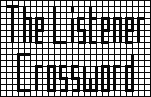## Listener Crossword 4075: Solution NotesBack to 2010 menu

## Square-bashing by Arden

### Detailed Solution

It is valuable to bear in mind that, since all entries are squares, no entry can end in 2, 3, 7 or 8.

 (1) 11ac (TIT) squares to a 2-digit answer so it must lie in the range 4 to 9, which makes T = 1, 2 or 3, and also places limits on I: if T>1 then I=1. 22dn (I+T) must also lie in this range so we cannot have T=2 and I=1. In the situation where T=3 and I=1, 16dn (TRYST) would have a minimum value of 360, which is too large for squaring to 5 digits. This fixes T. T = 1 (2) 20dn (TUT) must be at least 10 so U must be at least 10. This puts severe limits on R in 7ac (B+URR), which is less than 100, so R can only be 2 or 3. Also if R=3 then U=10 and B<10, which are too small for 4dn (BU/R); this fixes R. R = 2 (3) There are six clues that yield 2-digit squares (8ac, 11ac and 22dn), which must cover all the values in the range 4 to 9. 20dn (MR) has 3 digits, which limits M to no more than 15, and 11ac (M/R) makes M even: 8, 10, 12 or 14. 1dn (S²T) makes S at least 6, so 8ac (MR/S) forces M=12 with S=6, or M=14 with S=7. In either case MR/S = 4. (4) Now 22dn (ART/S) must be greater than 4 since all grid entries are different and 4 is already used. This gives no suitable value for A unless the first of the options in (3) is used. This establishes 11ac (M/R) as 6, which in turn forces 22dn (ART/S) to be 5 and A to be 15; the alternative 6, 18 is impossible since 6 is already used for 11ac. S = 6M = 12A = 15 (5) 8ac (AS/K) is now 90/K and must equal 7, 8 or 9, which are the only values giving a 2-digit square still available. This gives K. Now only 7 and 8 are left for 11ac (TIT) and 22dn (I+T), which gives I. K = 10I = 7 (6) 9ac (K+AY) now has two of its values known in K and A, both of which are multiples of 5, which makes K+AY a multiple of 5 and its square ---25 or ---00. This provides the final digit for a 4dn, one of whose entries is already known (SIR=84) as 7056. Hence BU/R must interact with K+AY and end with a zero (no square ends with a 2). (7) BU/R squared is a multiple of 10 so BU/R must be a multiple of 10, between 40 and 90. This severely limits sets of values for B and U, especially since U>10 from 20dn (TUT). We can have U=20 with B=4, 5, 8 or 9, or U=16 with B=5. (8) None of these pairs of values in 7ac (B+URR) agrees with 4dn (SIR). Hence B+URR goes with 4dn (BU/R) and TA+INT goes with SIR. TA+INT now ends with a zero, forcing N to be the only odd multiple of 5 available. N = 5 (9) Avoiding repeats in digit values and grid entries reduces the possibilities for B and U: B = 8 or 9. U = 20 (10) The two possible values for B give 7ac (B+URR) as 88 or 89, squared to 7744 or 7921. Neither of these values agrees with 2dn (TA+T) so they must correspond with RET, making this a 3-digit square with 7 or 9 in the middle. 20dn (MR) has already claimed 24² (=576), so only 196 and 676 are available, with E=7 or 13. Since I=7, we can deduce E and hence B. Also, 3dn is 666², which agrees only with 7ac (B+URR). E = 13B = 8 (11) 9ac (K+AY), known to have shape ---00, must now be --500, giving the value of Y. 9ac (C+AY) will only fit with one value of C. Y = 16C = 18 (12) 3dn (DI+SCS) gives D. 5dn (E+LITIST) can only have six digits with one value of L. 5dn (DON+OR) gives the value of O, since 8ac cannot start with 7. 6dn (BE+GINS) gives the value of G, since the other 5dn fixes both the 8ac entries. 10dn (IPS+O and P+URE) force the value of P. D = 9L = 3O = 17G = 14P = 19 (13) This leaves only F and H undecided, with 4 and 11 the possible values. 19ac (S+HIFT) can be filled in and the grid completed, with 614656 and 184041 as perfect squares to fit the bottom row. (14) This leaves the need to decide on the values of F and H and the order of the two sides. Both possibilities for F and H deliver a perfect square at 1ac that would fit, whichever way round the grid is arranged. Hence only the need to find suitable “words” for the bottom row can decide the matter. 184041 = 429², and 429 = 3.11.13 → L & F/H & E & (possibly) T. 614656 = 784², and 784 = 2.2.2.2.7.7 → some combination of R (2), F or H (4), B (8), Y (16), I (7), G (14) and (possibly) T. The obviously relevant words are LEFT (429) and RIGHT (784), which fix the correct order for the two halves of the grid, as well as F and H. F = 11H = 4
Back to 2010 menu